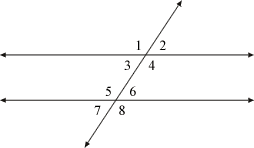index: click on a letter A B C D E F G H I J K L M N O P Q R S T U V W X Y Z A to Z index index: subject areas numbers & symbols sets, logic, proofs geometry algebra trigonometry advanced algebra & pre-calculus calculus advanced topics probability & statistics real world applications multimedia entrieswww.mathwords.com about mathwords website feedback

Alternate Interior Angles

In the drawing below, angles 3 and 6 are alternate interior angles, as are angles 4 and 5. Alternate interior angles are congruent. Formally, alternate interior angles are two interior angles which lie on different parallel lines and on opposite sides of a transversal.

 Parallel lines cut by a transversal